# Excel中SUBSTITUTE函数用法总结EXCEL手机版（内含百种各行模版）：点击查看

SUBSTITUTE函数的基础语法是：

`SUBSTITUTE(要替换的文本,旧文本,新文本,[替换第几个])`

## 1，将数据中的某个值替换为另一个值

C2输入公式，并向下复制填充：

`=SUBSTITUTE(B2,"二班","一班")`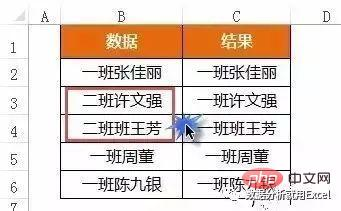## 2，隐藏手机号的中间5位

C2输入公式，并向下复制填充：

`=SUBSTITUTE(B2,MID(B2,4,5),"*****")`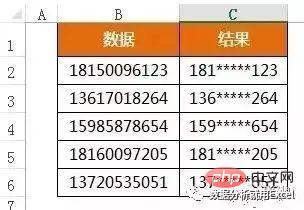## 3，对含单位的数据求和

B7输入公式：

`=SUMPRODUCT(SUBSTITUTE(B2:B6,"人",)*1)`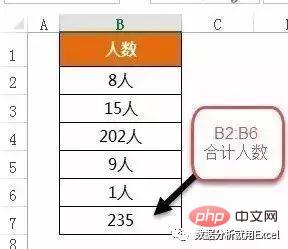## 4，数据分列，将B列数据按顿号进行分列

C2单元格输入公式横向拖动，并向下复制填充。

`=TRIM(MID(SUBSTITUTE(\$B2,"、",REPT(" ",100)),COLUMN(A1)*100-99,100))`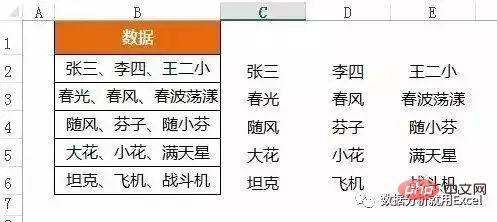REPT(” “,100)

SUBSTITUTE(\$B2,”、”,REPT(” “,100))

MID(SUBSTITUTE(\$B2,”、”,REPT(” “,100)),COLUMN(A1)*100-99,100)

## 5，混合文本中，计算人数个数B2公式：

`=LEN(A2)-LEN(SUBSTITUTE(A2,"、",))+1`

LEN(A2)取得A2字符串的长度。

LEN(SUBSTITUTE(A2,”、”,))+1，替换掉人名之间的间隔符，也就是顿号，再用LEN计算该值的长度，最后加1，是因为最后一个人名没有顿号。

## 6，混合文本中，计算数值最大值。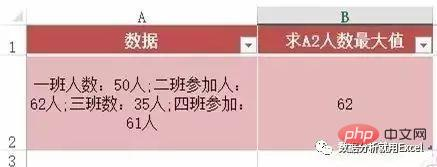B2数组公式：

`=MAX((SUBSTITUTE(A2,ROW(\$1:\$98),)<>A2)*ROW(1:98))`

SUBSTITUTE(A2,ROW(\$1:\$98),)<>A2

## 7，计算某个值在某个范围的最大连续次数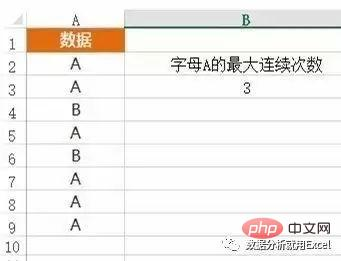B2数组公式：

`=MAX((SUBSTITUTE(PHONETIC(A2:A9),REPT("A",ROW(1:9)),)<>PHONETIC(A2:A9))*ROW(1:9))`

PHONETIC(A2:A9)

REPT(“A”,ROW(1:9))

`SUBSTITUTE(PHONETIC(A2:A9),REPT(“A”,ROW(1:9)),)<>PHONETIC(A2:A9)`1/1